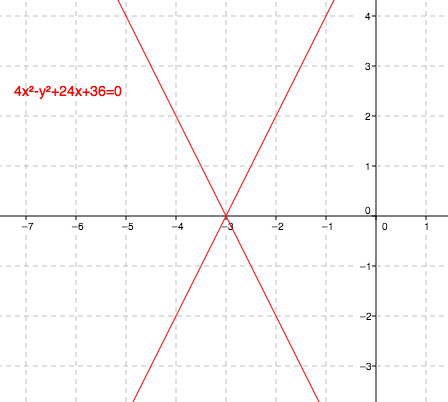### Home > A2C > Chapter 11 > Lesson 11.3.4 > Problem11-165

11-165.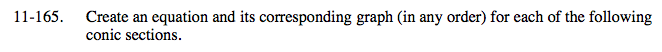For the following problems, use the general equation Ax2 + By2 + Dx + Ey + F = 0. Reminder: Uncheck the example before moving on to the next question.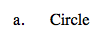In a circle with the center at (0, 0), A = B, and D = E = 0, and F < 0.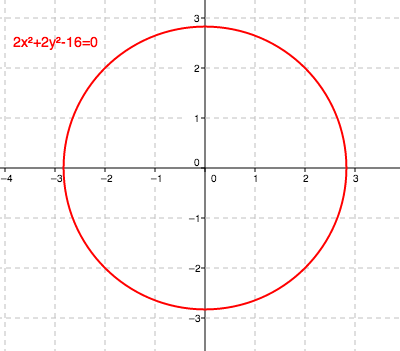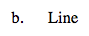In a linear equation, A = B = 0.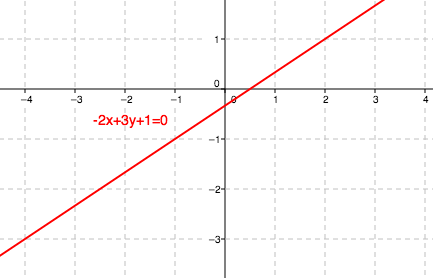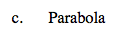In a sleeping parabola, A = 0 and B ≠ 0.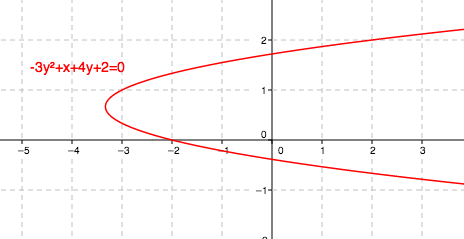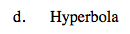In a horizontal hyperbola, A > 0, B < 0, and F < 0.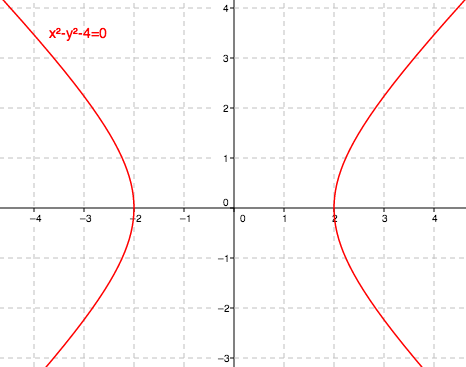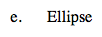In an ellipse, D = 0, E = 0, A ≠ 0, and B ≠ 0.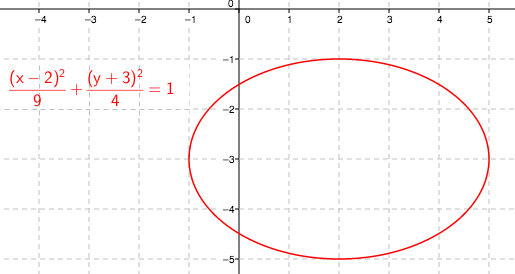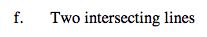Write the equations of two lines and multiply.